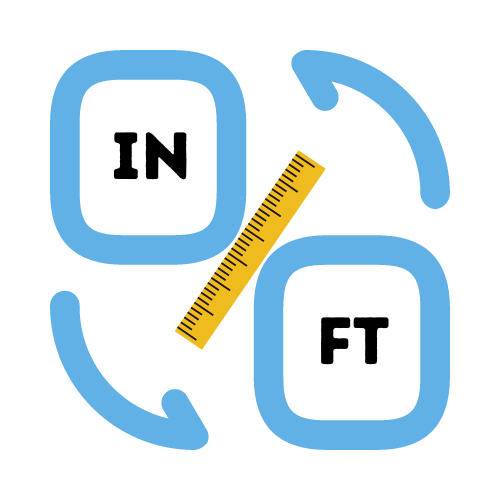# Feet to Inches Converter

## Convert feet to inches with this free conversion calculator

• Created by Emily Rodriguez
• Reviewed by Ramesh Agarwal

## How many inches are in a foot?

There are 12 inches in a foot. Knowing this makes it easy to convert between feet and inches. It is common practice to abbreviate the values of feet and inches when describing the length of an object or height of something. For example, we could describe our height as being 5 feet and 8 inches. In most cases this will be abbreviated to 5'8 (alternatively 4 feet 8) to make it easier to say within a conversation.

## What is the feet to inches formula?

Each foot has a length of 12 inches. An inch is equal to the length of 0.0833 feet. Once we know the corresponding length for each unit, we can calculate the length in either feet or inches quite easily. An example conversion of foot to inches: 10 foot to inches can be calculated as `10 * 12 = 120 inches`.

## How to use the feet to inches calculator

You can easily calculate the length in inches by entering the total length in feet using the feet to inches converter. The calculator will simply multiply the value (whole number) you enter by 12 to get the total length in inches.

To convert from feet to inches manually, follow these steps:

1. Take the number of feet and multiply the value by 12.
2. Add the remaining inches (ie. 5'10 would be 10 inches).
3. The two values combined is the total number of inches.

You can also convert feet and inches to inches by using the single apostrophe in the feet to inches calculator on this page. For example, enter 5'10 into the text field and press the calculate button. The conversion will give a result of `5'10 = 70 inches`.

## Feet to Inches Conversion Chart

If you need to convert feet to inches then these feet to inches charts should come in handy. There are times we we ask ourselves how many inches is 5 feet or how many inches is 6 feet. Using a feet to inches conversion chart makes these conversions really easy and fast.

### Feet to Inches Conversion Chart for Common Measurements

This feet to inches conversion table contains frequently used measurements for everyday values. Find the value in feet (left column) and the result in inches (right column).

Length in Feet Length in Inches
1 feet 12 inches
2 feet 24 inches
3 feet 36 inches
4 feet 48 inches
5 feet 60 inches
6 feet 72 inches
7 feet 84 inches
8 feet 96 inches
9 feet 108 inches
10 feet 120 inches
11 feet 132 inches
12 feet 144 inches
13 feet 156 inches
14 feet 168 inches
15 feet 180 inches
16 feet 192 inches
17 feet 204 inches
18 feet 216 inches
19 feet 228 inches
20 feet 240 inches

### Feet to Inches Conversion Chart for Height

Convert from feet to inches with this easy to use feet to inches chart for height. Find the height measurement in feet (left column) and the result in inches (right column).

Feet Inches
4'10 58 inches
4'11 59 inches
5' 60 inches
5'1 61 inches
5'2 62 inches
5'3 63 inches
5'4 64 inches
5'5 65 inches
5'6 66 inches
5'7 67 inches
5'8 68 inches
5'9 69 inches
5'10 70 inches
5'11 71 inches
6' 72 inches
6'1 73 inches
6'2 74 inches
6'3 75 inches
6'4 76 inches
6'5 77 inches